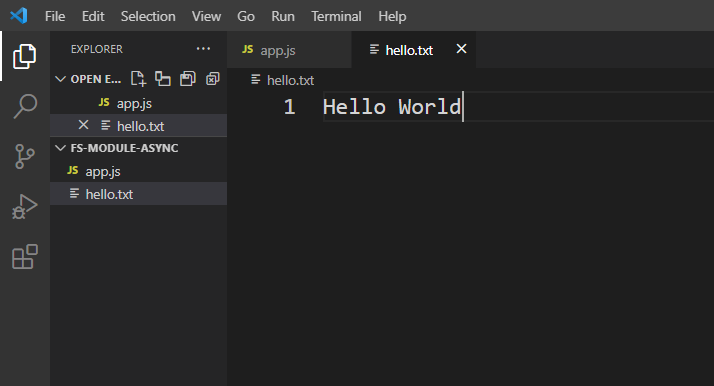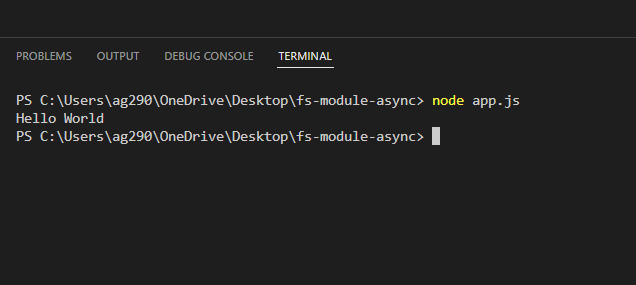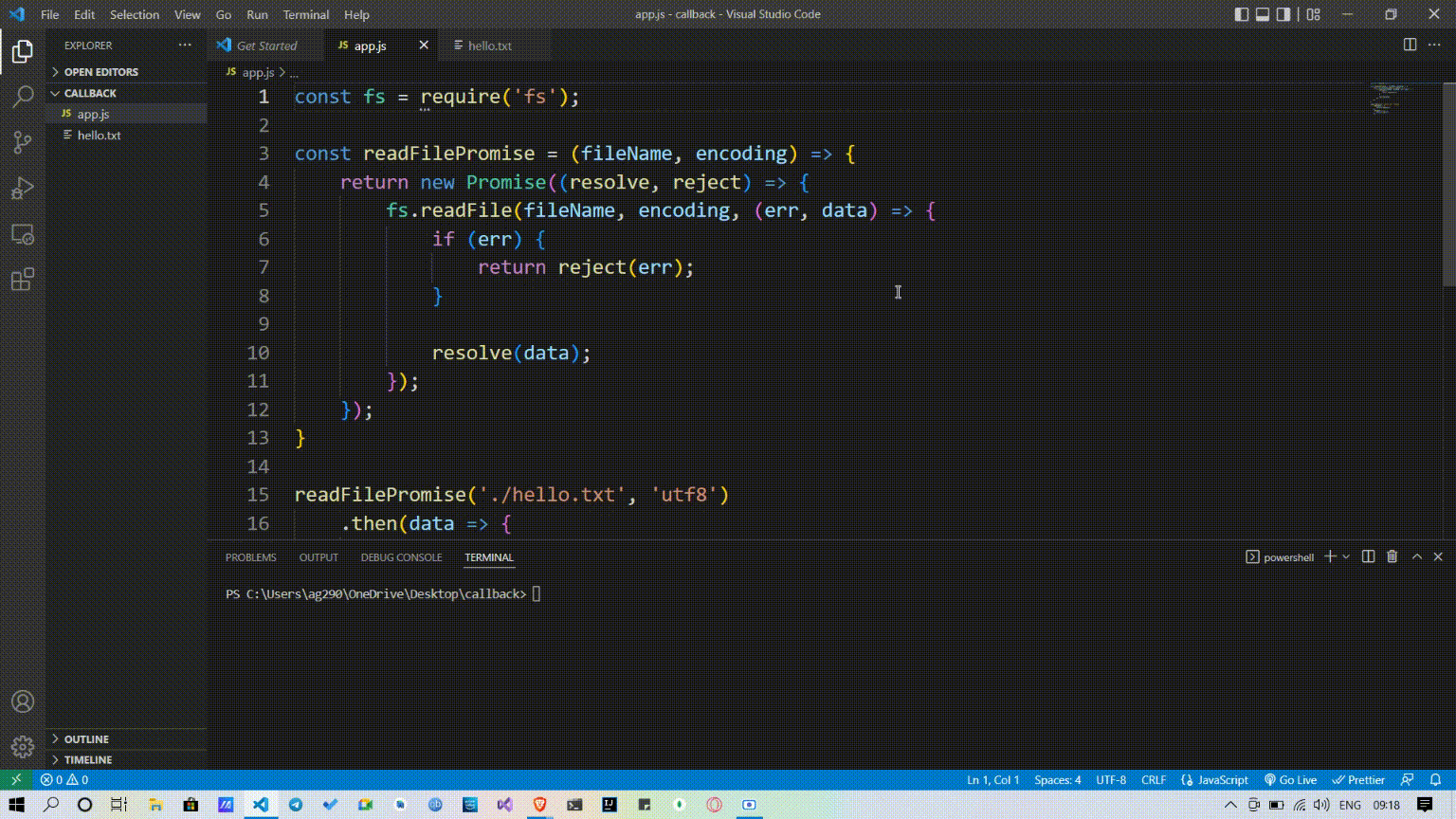# How to convert function call with two callbacks promise in Node.js ?

• Last Updated : 19 May, 2022

Promise: Promise is used to handle the result, if it gets the required output then it executes the code of then block, and if it gets the error then it executes the code of the catch block.

A promise looks like this –

```function()
.then(data => {

// After promise is fulfilled
console.log(data);
})
.catch(err => {

// After promise is rejected
console.error(err);
});```

Callback: A callback is the last argument of a function. It will be executed to do something with the values that we get from the function. A function call with two callbacks looks like this

```fs.readFile('./hello.txt', ,'utf8', (err, data) => {
if (err) {
console.error(err);
return;
}
console.log(data);
});```

This is a readFile function of the File System Module. It is used to read the data of a file. readFile function can call with two parameters: File Path, File Encoding, and callbacks. File-path is the path where your file is present & File Encoding is the encoding to that file, by default it is “utf8”. Inside callbacks, check for the error, if error then logs the error, if no then log the result.

Let’s run this function first: Make sure that you have created a file “hello.txt” with some content on it in this case it is ‘Hello  World’.Example:

## Javascript

 `const fs = require(``'fs'``);`` ` `fs.readFile(``'./hello.txt'``, ,``'utf8'``, (err, data) => {``     ``if` `(err) {``         ``console.error(err);``               ``return``;``     ``}``     ``console.log(data);``});`

Output:let’s convert this into a promise.

Mechanism: For converting this readFile function into a promise, we have to make a new function let’s call it readFilePromise with two arguments fileName & encoding. Inside this function, we return a new promise, and this promise uses the readFile function inside it. If the readFile function returns the required output then it resolves the promise, which means it returns the data as a promise, and if the function doesn’t return the required output then it rejects the promise which means it returns an error instead of data.

```const readFile = (fileName, encoding) => {
return new Promise((resolve, reject) => {
fs.readFile(fileName, encoding, (err, data) => {
if (err) {
return reject(err);
}
resolve(data);
});
});
}```

Complete Code:

## Javascript

 `const fs = require(``'fs'``);`` ` `const readFilePromise = (fileName, encoding) => {``    ``return` `new` `Promise((resolve, reject) => {``        ``fs.readFile(fileName, encoding, (err, data) => {``            ``if` `(err) {``                ``return` `reject(err);``            ``}`` ` `            ``resolve(data);``        ``});``    ``});``}`` ` `readFilePromise(``'./hello.txt'``, ``'uft8'``)``    ``.then(data => {``        ``console.log(data);``    ``})``    ``.``catch``(err => {``        ``console.log(err);``    ``});`

Step to run the application: Open the terminal and type the following command.

`node app.js`

Output:My Personal Notes arrow_drop_up